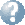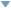Online Reference Dictionary, Encyclopedia & moreby: WordStarts with Ends withMentionsteaching:topics:calculus:introduction

## ideas to consider as you start Calculus

The calculus you are learning is called Infinitesimal Calculus. Yes — there are others! Mathematics is a vast field and we only just touch the surface in school. Mostly 17th century maths, with occasional more modern refinements and notation.

Calculus is concerned with differentiation and integration.

Differentiation is about finding the ratio between two vanishingly small quantities,

Integration is about adding up an infinite number of vanishingly narrow slices of something.

Infinitessimals is the name for these vanishingly tiny things

They are a way to work with the slopes of curves and the areas under them. These properties are often very useful when the curve is describing some real-world system, some pattern we observe around us. We will be finding functions describing these values. We will be making mathematical models that can be used to describe things we observe around us.

Dealing properly with ideas like "infinitessimals" or “infinite” is difficult, we need to be quite careful how we do it. They do not behave like numbers, so we cannot just treat them like a new kind of number. Look at Sums of Sequences and Limits for a discussion about working with infinitessimals. Historically following these ideas is what led to Calculus. That path was very contraversial. It took a few generations of dedicated effort by many mathematicians to get there. Since then mathematicians have worked to make it more general and more rigorous, it has many very important applications.

Calculus is a powerful method when considering things that have changing magnitudes which are measured in continuous rather than discrete units — especially where we can find functions that describe some relationship between these measurements. We use it in practical applications to help understand and predict behaviours of systems in Physics, Biology, Meteorlogy, business, Economics, computing, society, most science and much analysis when trying to develop policy and manage organisations and society. It is also a very important part of pure mathematics, part of the way we develop mathematical thinking and new mathematical ideas — including new ways to model (that is describe, predict and discuss) the behavior of things we see around us.

### Motion

We start exploring calculus (just like they did in the 17th century, while inventing it) by trying to describe the way objects move. Seconds and metres are both measurements that are not restricted to whole numbers. Length and time (at least on any scale we are concerned with here!!) can take any real-number value. Speed is a number saying how far an object goes during each unit of time — a sprinter running at $10\,$m/s moves 10 metres every second. Acceleration is a measure of how quickly speed is changing. We discovered that this last property is much more regular, that there are very specific rules that natural objects seem to obey concerning acceleration.

We know how to find the average speed during a journey: $\quad\dfrac{\texttt{distance travelled}}{\texttt{time taken}},\quad$

but then how do we find the speed at some instant, when it might be continuously changing? In that instant 0m was travelled during 0s but we cannot just do $0\div0$, it will not give us a sensible answer. Instead we could consider the rate of change of position (over time!), or we could consider a graph we draw with position (that is distance from some fixed place) on the vertical axis and time (since some fixed start time) on the horizontal axis. Then we see that speed is the same as the slope of a line on this graph — the \ tt{rise}\ \  is the same as change in position, which is the distance travelled and the \ tt{run}\ \  is the time taken for that to happen.

So in this case the slope, $\quad\dfrac{\tt rise}{\tt run},\quad$ is the velocity of a moving object described by that line.

Speed is a number — when we want to consider direction as well we speak of velocity, which is a vector. With only one dimension (say height) like in the graph described we can use Real numbers, positive or negative depending on direction, instead of a vector.

So we have a model for the motion of an object presented as a graph, and we see that slope in this context is analagous to velocity. We can easily calculate the slope of a straight line, but how do we calculate this property of a curve? This will be our first exploration in calculus.

There are three notations for Calculus, they come from quite different mathematical perspectives. You should become familiar with each of them, they are used in different contexts. The story behind these very different ways of writing derivatives might help you get a better idea of the different ways we work with them.

#### Before we begin

lets look at a closely related question about motion that remained unanswered for 2000 years …
a paradox, and sums of sequences